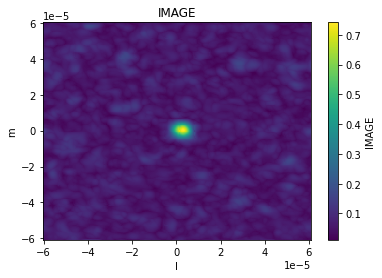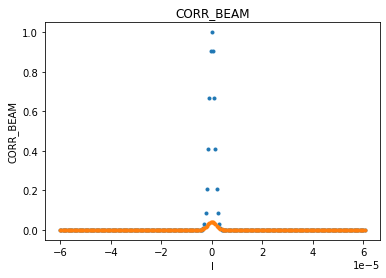# Images¶

An overview of the image data structure and manipulation.

This walkthrough is designed to be run in a Jupyter notebook on Google Colaboratory. To open the notebook in colab, go here

[ ]:

# Installation
# for this demonstration we will use the data from the ALMA First Look at Imaging CASAguide
import os, warnings
warnings.simplefilter("ignore", category=RuntimeWarning)  # suppress warnings about nan-slices
print("installing cngi (takes a few minutes)...")
os.system("apt-get install libgfortran3")
os.system("pip install cngi-prototype==0.0.57")

os.system("tar -xvf sis14_twhya_calibrated_flagged.ms.tar")

print('complete')

installing cngi (takes a few minutes)...
complete


## Initialize the Environment¶

InitializeFramework instantiates a client object (does not need to be returned and saved by caller). Once this object exists, all Dask objects automatically know to use it for parallel execution.

>>> from cngi.direct import InitializeFramework
>>> client = InitializeFramework(workers=4, memory='2GB')
>>> print(client)
<Client: 'tcp://127.0.0.1:33227' processes=4 threads=4, memory=8.00 GB>


Omitting this step will cause the subsequent Dask dataframe operations to use the default built-in scheduler for parallel execution (which can actually be faster on local machines anyway)

Google Colab doesn’t really support the dask.distributed environment particularly well, so we will let Dask use its default scheduler.

## Create a CASA Image¶

First we need to create a CASA image by calling CASA6 tclean on the CASAguide MS

Pro tip: you can see the files being created by expanding the left navigation bar in colab (little arrow on top left) and going to “Files”

[ ]:

from casatasks import tclean
tclean(vis='sis14_twhya_calibrated_flagged.ms', imagename='sis14_twhya_calibrated_flagged', field='5', spw='',
specmode='cube', deconvolver='hogbom', nterms=1, imsize=[250,250], gridder='standard', cell=['0.1arcsec'],
nchan=10, weighting='natural', threshold='0mJy', niter=5000, interactive=False, savemodel='modelcolumn',
print('complete')

complete


## Convert Image to Xarray/Zarr¶

tclean produces an image along with a number of supporting products (residual, pb, psf, etc) in their own separate directories.

CNGI uses an xarray data model and the zarr storage format which is capable of storing all image products (of the same shape) together.

Note that the .mask image product will be renamed to ‘automask’ in the xarray data model and ‘mask’ will hold the mask pixel values extracted from the .image.

[ ]:

from cngi.conversion import convert_image

convert_image('sis14_twhya_calibrated_flagged.image');

converting Image...
compatible components:  ['image', 'pb', 'psf', 'residual', 'mask', 'model', 'sumwt']
separate components:  []
processed image size [250 250   1  10] in 1.8118241 seconds


## Open and Inspect Image Data¶

Open the zarr format image and print its contents. Note that the zarr file has all the image products in it (rather than separate directories)

[ ]:

from cngi.dio import read_image

# returns an xarray dataset representation of the specified zarr directory


Within the xarray dataset image, we can see a very clear definition of the image properties. The Dimensions section holds the size of the image, while the Coordinates section defines the values of each dimension. The actual image (and supporting products) are stored in the Data variables section. Lastly, the Attributes section holds the remaining metadata.

Note that the variables image, automask, mask, model, pb, psf, and residual all share the same dimensions of 250x250x1x10 while the variable sumwt is a subset of dimensions 1x10

[ ]:

image_xds

xarray.Dataset
• chan: 10
• d0: 250
• d1: 250
• pol: 1
• chan
(chan)
float64
3.725e+11 3.725e+11 ... 3.725e+11
array([3.725200e+11, 3.725206e+11, 3.725212e+11, 3.725219e+11, 3.725225e+11,
3.725231e+11, 3.725237e+11, 3.725243e+11, 3.725249e+11, 3.725255e+11])
• declination
(d0, d1)
float64


Array  Chunk

Bytes  500.00 kB   500.00 kB
Shape  (250, 250)   (250, 250)
Type  float64  numpy.ndarray

250
250


• pol
(pol)
float64
1.0
array([1.])
• right_ascension
(d0, d1)
float64


Array  Chunk

Bytes  500.00 kB   500.00 kB
Shape  (250, 250)   (250, 250)
Type  float64  numpy.ndarray

250
250


(d0, d1, chan, pol)
bool


Array  Chunk

Bytes  625.00 kB   62.50 kB
Shape  (250, 250, 10, 1)   (250, 250, 1, 1)
Type  bool  numpy.ndarray

250
1

1
10
250


• IMAGE
(d0, d1, chan, pol)
float64


Array  Chunk

Bytes  5.00 MB   500.00 kB
Shape  (250, 250, 10, 1)   (250, 250, 1, 1)
Type  float64  numpy.ndarray

250
1

1
10
250


(d0, d1, chan, pol)
bool


Array  Chunk

Bytes  625.00 kB   62.50 kB
Shape  (250, 250, 10, 1)   (250, 250, 1, 1)
Type  bool  numpy.ndarray

250
1

1
10
250


• MODEL
(d0, d1, chan, pol)
float64


Array  Chunk

Bytes  5.00 MB   500.00 kB
Shape  (250, 250, 10, 1)   (250, 250, 1, 1)
Type  float64  numpy.ndarray

250
1

1
10
250


• PB
(d0, d1, chan, pol)
float64


Array  Chunk

Bytes  5.00 MB   500.00 kB
Shape  (250, 250, 10, 1)   (250, 250, 1, 1)
Type  float64  numpy.ndarray

250
1

1
10
250


• PSF
(d0, d1, chan, pol)
float64


Array  Chunk

Bytes  5.00 MB   500.00 kB
Shape  (250, 250, 10, 1)   (250, 250, 1, 1)
Type  float64  numpy.ndarray

250
1

1
10
250


• RESIDUAL
(d0, d1, chan, pol)
float64


Array  Chunk

Bytes  5.00 MB   500.00 kB
Shape  (250, 250, 10, 1)   (250, 250, 1, 1)
Type  float64  numpy.ndarray

250
1

1
10
250


• SUMWT
(chan, pol)
float64


Array  Chunk

Bytes  80 B   8 B
Shape  (10, 1)   (1, 1)
Type  float64  numpy.ndarray

1
10


• axisunits :
commonbeam :
[0.6530517935752869, 0.5046663284301758, -66.00911712646484]
commonbeam_units :
['arcsec', 'arcsec', 'deg']
date_observation :
2012/11/19/07
direction_reference :
j2000
imagetype :
Intensity
incr :
[-4.84813681109536e-07, 4.84813681109536e-07, 1.0, 610330.1590576172]
object_name :
tw hya
observer :
cqi
pointing_center :
11
rest_frequency :
3.7265e+11 hz
restoringbeam :
[[[0.6530517935752869, 0.5046663284301758, -66.00911712646484]], [[0.6530517935752869, 0.5046663284301758, -66.00911712646484]], [[0.6530508399009705, 0.5046656131744385, -66.00914764404297]], [[0.653049647808075, 0.5046647787094116, -66.0091552734375]], [[0.6530488133430481, 0.5046640038490295, -66.0091552734375]], [[0.6530477404594421, 0.5046632885932922, -66.00917053222656]], [[0.6530467867851257, 0.5046625137329102, -66.00917053222656]], [[0.6530455946922302, 0.5046617388725281, -66.00917053222656]], [[0.6530444622039795, 0.5046609044075012, -66.00918579101562]], [[0.6530434489250183, 0.5046601295471191, -66.00914764404297]]]
spectral__reference :
lsrk
telescope :
alma
telescope_position :
[2.22514e+06m, -5.44031e+06m, -2.48103e+06m] (itrf)
unit :
Jy/beam
velocity__type :

The image has both cartesian pixel coordinates (d0, d1) and spherical world coordinates (right_ascension, declination).

## Preview Image¶

We can quickly spot check image contents. This is also handy later on during image manipulation and analysis. Most image data is four-dimensional, so the plotting routine will collapse extra dimensions by taking the max (this is more robust than mean).

The image can be plotted using its spherical world coordinates or cartesian pixel indices

[ ]:

from cngi.image import implot

# just channel 5
implot(image_xds.IMAGE, axis=['right_ascension', 'declination'], chans=5)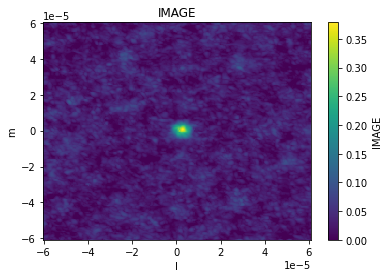[ ]:

# max of all channels, with cartesian pixel indices
implot(image_xds.IMAGE, axis=['d0', 'd1'], chans=None)Other image data products are plotted the same way

[ ]:

implot(image_xds.RESIDUAL, chans=5)The plotting function has the same capabilities as the visibility routine, so we can also make scatter plots across coordinates and dimensions using the axis parameter. Other dimensions are collapsed by taking the max.

[ ]:

implot(image_xds.IMAGE, axis='d0')Looking across channels, it is clear that channel 0 is empty. This is why the above plot across all channels is rescaled to a min value of 0.

[ ]:

implot(image_xds.IMAGE, axis='chan')## Basic Manipulation¶

Many image operations can be easily done directly on the image xarray dataset

Example 1: imsubimage - copy all or part of an image to a new image

[ ]:

# selection by coordinate values
image_xds2 = image_xds.where( (image_xds.right_ascension > 2.887905) & (image_xds.right_ascension < 2.887935) &
(image_xds.declination > -0.60573) & (image_xds.declination < -0.60570), drop=True )
implot(image_xds2.IMAGE, chans=5)

# selection by pixel indices (50x50 pixel square)
# note that this same method works for any other dimension (stokes, channels, etc)
image_xds2 = image_xds.isel(d0=range(85,135), d1=range(100,150))
implot(image_xds2.IMAGE, chans=5)Example 2: imtrans - reorders (transposes) the axes in the input image to the specified order

This can be done on the entire dataset, or just one variable in the dataset. Here we will do it on the whole thing which is probably best if you want to preserve your regions and masks (explained later). This will generally not affect the preview plot since it selects axes by name rather than index. That is one advantage of xarray over numpy.

[ ]:

image_xds2 = image_xds.transpose('chan','d1','d0','pol')
print('Before\n', image_xds.data_vars)
print('\nAfter\n', image_xds2.data_vars)

Before
Data variables:
IMAGE     (d0, d1, chan, pol) float64 dask.array<chunksize=(250, 250, 1, 1), meta=np.ndarray>
MODEL     (d0, d1, chan, pol) float64 dask.array<chunksize=(250, 250, 1, 1), meta=np.ndarray>
PB        (d0, d1, chan, pol) float64 dask.array<chunksize=(250, 250, 1, 1), meta=np.ndarray>
PSF       (d0, d1, chan, pol) float64 dask.array<chunksize=(250, 250, 1, 1), meta=np.ndarray>
RESIDUAL  (d0, d1, chan, pol) float64 dask.array<chunksize=(250, 250, 1, 1), meta=np.ndarray>
SUMWT     (chan, pol) float64 dask.array<chunksize=(1, 1), meta=np.ndarray>

After
Data variables:
IMAGE     (chan, d1, d0, pol) float64 dask.array<chunksize=(1, 250, 250, 1), meta=np.ndarray>
MODEL     (chan, d1, d0, pol) float64 dask.array<chunksize=(1, 250, 250, 1), meta=np.ndarray>
PB        (chan, d1, d0, pol) float64 dask.array<chunksize=(1, 250, 250, 1), meta=np.ndarray>
PSF       (chan, d1, d0, pol) float64 dask.array<chunksize=(1, 250, 250, 1), meta=np.ndarray>
RESIDUAL  (chan, d1, d0, pol) float64 dask.array<chunksize=(1, 250, 250, 1), meta=np.ndarray>
SUMWT     (chan, pol) float64 dask.array<chunksize=(1, 1), meta=np.ndarray>


Example 3: imcollapse - collapse an image along a specified axis or set of axes of N pixels into a single pixel on each specified axis.

Again, this can be done on the entire dataset, or just one variable in the dataset. Refer to the xarray documentation for supported aggregation functions, or write your own and call reduce. This will generally break the preview plot since it is expecting a 4-D cube with ra, dec, stokes, and frequency axes

[ ]:

# one dimension collapse
image_xds2 = image_xds.sum(dim='chan')
print('One dimension collapse\n', image_xds2.data_vars)

# multi-dimension collapse
image_xds2 = image_xds.mean(dim=['d0','d1'])
print('\nMulti-dimension collapse\n', image_xds2.data_vars)


One dimension collapse
Data variables:
IMAGE     (d0, d1, pol) float64 dask.array<chunksize=(250, 250, 1), meta=np.ndarray>
MODEL     (d0, d1, pol) float64 dask.array<chunksize=(250, 250, 1), meta=np.ndarray>
PB        (d0, d1, pol) float64 dask.array<chunksize=(250, 250, 1), meta=np.ndarray>
PSF       (d0, d1, pol) float64 dask.array<chunksize=(250, 250, 1), meta=np.ndarray>
RESIDUAL  (d0, d1, pol) float64 dask.array<chunksize=(250, 250, 1), meta=np.ndarray>

Multi-dimension collapse
Data variables:
IMAGE     (chan, pol) float64 dask.array<chunksize=(1, 1), meta=np.ndarray>
MODEL     (chan, pol) float64 dask.array<chunksize=(1, 1), meta=np.ndarray>
PB        (chan, pol) float64 dask.array<chunksize=(1, 1), meta=np.ndarray>
PSF       (chan, pol) float64 dask.array<chunksize=(1, 1), meta=np.ndarray>
RESIDUAL  (chan, pol) float64 dask.array<chunksize=(1, 1), meta=np.ndarray>
SUMWT     (chan, pol) float64 dask.array<chunksize=(1, 1), meta=np.ndarray>


Both regions and masks are stored as boolean arrays in the same xarray dataset alongside the rest of the image components. They always share the same dimensions as the image.

They are both now treated as the same thing, so a value of 0 means “discard this pixel” and a value of 1 means “keep this pixel” regardless of whether it is a mask or a region. In fact, any xarray data variable of boolean type can be used as a region/mask, there is nothing special about the names.

Regions/masks can be set across any dimension, so they can be per-channel and per-stokes as well as the spatial dimensions.

### Regions¶

First lets create a couple examples:

[ ]:

from cngi.image import region

# region 1 is an ra/dec box across all channels
image_xds2 = region(image_xds, 'REGION1', ra=[2.887905, 2.887935], dec=[-0.60573, -0.60570])

# lets examine what it looks like
implot(image_xds2.REGION1, chans=5)

# and combined with our image
implot(image_xds2.IMAGE.where(image_xds2.REGION1), chans=5)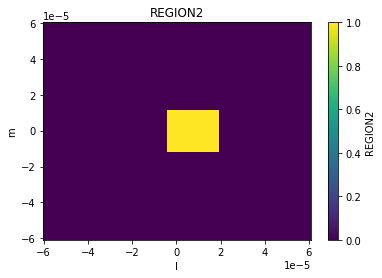[ ]:

# region 2 is a pixel box across channels 3, 4 and 5
image_xds3 = region(image_xds2, 'REGION2', pixels=[[85,100],[135,150]], channels=[3,4,5])

# observe the change in behavior across channels
implot(image_xds3.REGION2, chans=5)
implot(image_xds3.REGION2, chans=6)
implot(image_xds3.IMAGE.where(image_xds3.REGION2), chans=[5,6])Regions are just data variables in the xarray dataset. So we can manipulate them the same way we can any other variable.

[ ]:

image_xds3.data_vars

Data variables:
IMAGE     (d0, d1, chan, pol) float64 dask.array<chunksize=(250, 250, 1, 1), meta=np.ndarray>
MODEL     (d0, d1, chan, pol) float64 dask.array<chunksize=(250, 250, 1, 1), meta=np.ndarray>
PB        (d0, d1, chan, pol) float64 dask.array<chunksize=(250, 250, 1, 1), meta=np.ndarray>
PSF       (d0, d1, chan, pol) float64 dask.array<chunksize=(250, 250, 1, 1), meta=np.ndarray>
RESIDUAL  (d0, d1, chan, pol) float64 dask.array<chunksize=(250, 250, 1, 1), meta=np.ndarray>
SUMWT     (chan, pol) float64 dask.array<chunksize=(1, 1), meta=np.ndarray>
REGION1   (d0, d1, chan, pol) bool dask.array<chunksize=(250, 250, 1, 1), meta=np.ndarray>
REGION2   (d0, d1, chan, pol) bool dask.array<chunksize=(250, 250, 1, 1), meta=np.ndarray>


Let’s combine the first two regions in to a new one (doesn’t need to be contiguous, although it is here). It is just a logical OR of two boolean arrays (obviously you could do an AND, XOR, or whatever else).

Just keep in mind that these are 4-D arrays, not 2-D.

[ ]:

region3 = image_xds3.REGION1 | image_xds3.REGION2
image_xds4 = image_xds3.assign(dict([('REGION3', region3)]))

implot(image_xds4.REGION3, chans=5)
implot(image_xds4.IMAGE.where(image_xds4.REGION3), chans=5)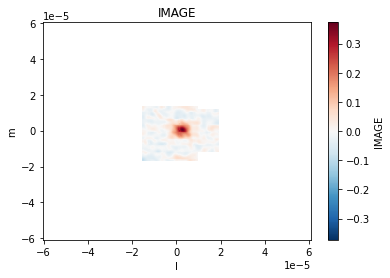Masks are just like regions but with inverse logic used to set them. Here is the same code again using calls to mask instead of region.

[ ]:

from cngi.image import mask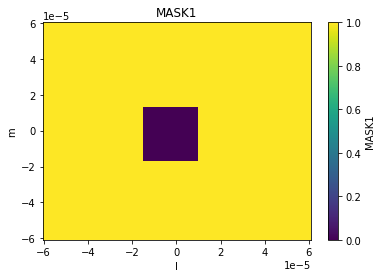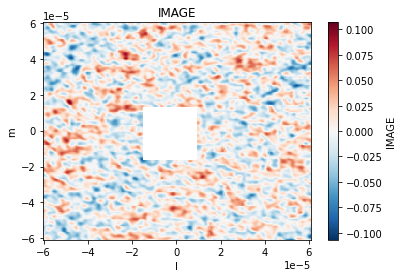These actually look a lot more like regions than masks, but that’s the terminology that was used. Again, they are the same thing anyway

[ ]:

implot(image_xds2.AUTOMASK, chans=5)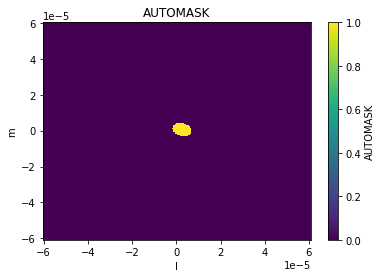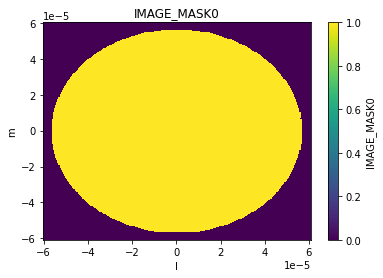### Manipulation with Regions and Masks¶

All of the previously discussed image manipulation techniques can be done with regions and masks applied. Just subselect the pixels within the region (outside the mask) first. This is done with dataset.where(…, drop=True)

Here is example 3 again with a region applied.

Example 3: imcollapse - collapse an image along a specified axis or set of axes of N pixels into a single pixel on each specified axis.

[ ]:

# full image collapse
image_xds2 = image_xds.mean(dim=['d0','d1'])
print('Mean pixel values by channel : ', image_xds2.isel(pol=0).IMAGE.values, '\n')

# apply deconvolve region from auto-masking
implot(image_xds2.IMAGE)

# collapse just the region
image_xds2 = image_xds2.mean(dim=['d0','d1'])
print('\nWith deconvolve mask applied : ', image_xds2.isel(pol=0).IMAGE.values)

Mean pixel values by channel :  [0.         0.0009337  0.00062645 0.00089679 0.00076623 0.00075292
0.00072544 0.00098671 0.00082494 0.00076202]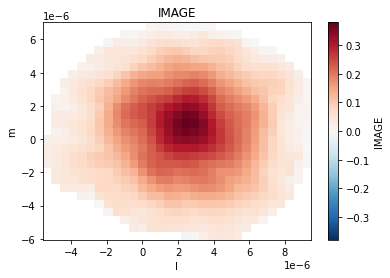With deconvolve mask applied :  [0.10398341 0.09321037 0.11130808 0.18811812 0.18880713 0.11977938
0.12009657 0.09283661 0.10214104]


## Beams and Smoothing¶

Smoothing an image involves creating and convolving beams of appropriate parameters to achieve the desired target properties in the image.

### Creating Beams¶

Beams may be created as additional products in the image xarray Dataset. They may also be present already in parameterized form inside the attributes section.

First lets expand the parameterized per-plane beams defined in the xds attributes section. This beam is defined across the channels and polarization axes.

[ ]:

from cngi.image import gaussianbeam

print('restoring beam parameters (chan 5) : ', image_xds.restoringbeam)

image_xds2 = gaussianbeam(image_xds, source='restoringbeam', name='RESTORING_BEAM')

implot(image_xds2.RESTORING_BEAM)
print('restoring beam dimensions: ', image_xds2.RESTORING_BEAM.shape)

restoring beam parameters (chan 5) :  [[0.6530477404594421, 0.5046632885932922, -66.00917053222656]]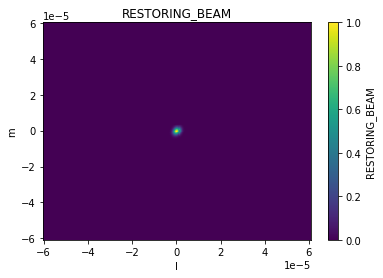restoring beam dimensions:  (250, 250, 10, 1)


Now lets create a larger beam from our own parameters. This one has only spatial dimensions.

[ ]:

image_xds2 = gaussianbeam(image_xds2, source=[1., 1., 30], name='BEAM')

implot(image_xds2.RESTORING_BEAM, 'd0', drawplot=False)
implot(image_xds2.BEAM, 'd0', overplot=True)
print('custom beam dimensions: ', image_xds2.BEAM.shape)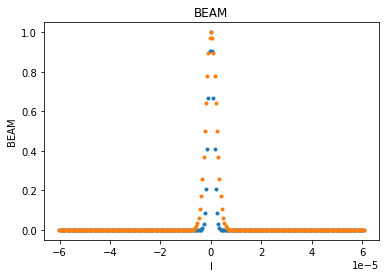custom beam dimensions:  (250, 250)


### Smoothing with Beams¶

The smooth function can take an existing beam and convolve it across the image to produce a new output image.

[ ]:

from cngi.image import smooth

# smooth with the restoring beam
image_xds3 = smooth(image_xds2, kernel='RESTORING_BEAM')

print('original image')
implot(image_xds2.IMAGE)

print('smooth image')
implot(image_xds3.IMAGE)

original image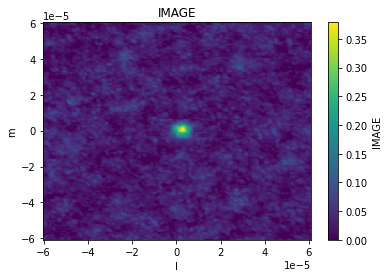smooth image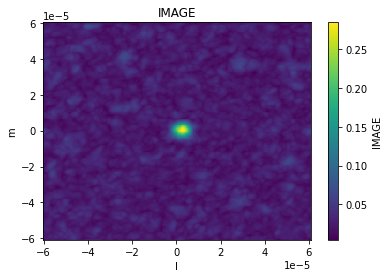[ ]:

# smooth with our larger custom beam
image_xds4 = smooth(image_xds2, kernel='BEAM')

implot(image_xds.IMAGE, axis='d0', chans=5, drawplot=False)
implot(image_xds3.IMAGE, axis='d0', chans=5, overplot=True, drawplot=False)
implot(image_xds4.IMAGE, axis='d0', chans=5, overplot=True)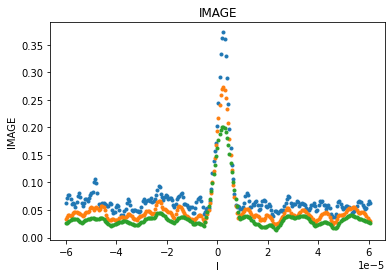If the image has already been smoothed by a previous beam, the smooth function can take the parameters of a new desired beam and construct the appropriate correcting beam to produce that target. The correcting beam used for smoothing is saved in the output xarray Dataset.

[ ]:

image_xds3 = smooth(image_xds2, kernel='gaussian', size=(1., 1., 60.), current=(0.65305, 0.50467, -66.), name='CORR_BEAM')

implot(image_xds3.IMAGE)
implot(image_xds3.RESTORING_BEAM, axis='d0', chans=5, drawplot=False)
implot(image_xds3.CORR_BEAM, axis='d0', overplot=True)